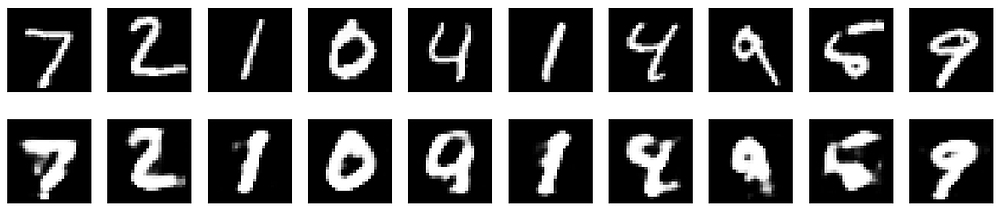# Implementing an Autoencoder in PyTorch

Source: Deep Learning on Medium# Autoencoder

Since the linked article above already explains what is an autoencoder, we will only briefly discuss what it is.

An autoencoder is a type of neural network that finds the function mapping the features x to itself. This objective is known as reconstruction, and an autoencoder accomplishes this through the following process: (1) an encoder learns the data representation in lower-dimension space, i.e. extracting the most salient features of the data, and (2) a decoder learns to reconstruct the original data based on the learned representation by the encoder.

Mathematically, process (1) learns the data representation z from the input features x, which then serves as an input to the decoder.

Then, process (2) tries to reconstruct the data based on the learned data representation z.

The encoder and the decoder are neural networks that build the autoencoder model, as depicted in the following figure,

To simplify the implementation, we write the encoder and decoder layers in one class as follows,

Explaining some of the components in the code snippet above,

• The `torch.nn.Linear` layer creates a linear function (`θx + b`), with its parameters initialized (by default) with He/Kaiming uniform initialization, as it can be confirmed here. This means we will call an activation/non-linearity for such layers.
• The `in_features` parameter dictates the feature size of the input tensor to a particular layer, e.g. in `self.encoder_hidden_layer`, it accepts an input tensor with the size of `[N, input_shape]` where `N` is the number of examples, and `input_shape` is the number of features in one example.
• The `out_features` parameter dictates the feature size of the output tensor of a particular layer. Hence, in the `self.decoder_output_layer`, the feature size is `kwargs["input_shape"]`, denoting that it reconstructs the original data input.
• The `forward()` function defines the forward pass for a model, similar to `call` in `tf.keras.Model`. This is the function invoked when we pass input tensors to an instantiated object of a `torch.nn.Module` class.

To optimize our autoencoder to reconstruct data, we minimize the following reconstruction loss,

We instantiate an autoencoder class, and move (using the `to()` function) its parameters to a `torch.device`, which may be a GPU (`cuda` device, if one exists in your system) or a CPU (lines 2 and 6 in the code snippet below).

Then, we create an optimizer object (line 10) that will be used to minimize our reconstruction loss (line 13).

For this article, let’s use our favorite dataset, MNIST. In the following code snippet, we load the MNIST dataset as tensors using the `torchvision.transforms.ToTensor()` class. The dataset is downloaded (`download=True`) to the specified directory (`root=<directory>`) when it is not yet present in our system.

After loading the dataset, we create a `torch.utils.data.DataLoader` object for it, which will be used in model computations.

Finally, we can train our model for a specified number of epochs as follows,

In our data loader, we only need to get the features since our goal is reconstruction using autoencoder (i.e. an unsupervised learning goal). The features loaded are 3D tensors by default, e.g. for the training data, its size is `[60000, 28, 28]`. Since we defined our `in_features` for the encoder layer above as the number of features, we pass 2D tensors to the model by reshaping `batch_features` using the `.view(-1, 784)` function (think of this as `np.reshape()` in NumPy), where 784 is the size for a flattened image with 28 by 28 pixels such as MNIST.

At each epoch, we reset the gradients back to zero by using `optimizer.zero_grad()`, since PyTorch accumulates gradients on subsequent passes. Of course, we compute a reconstruction on the training examples by calling our model on it, i.e. `outputs = model(batch_features)`. Subsequently, we compute the reconstruction loss on the training examples, and perform backpropagation of errors with `train_loss.backward()` , and optimize our model with `optimizer.step()` based on the current gradients computed using the `.backward()` function call.

To see how our training is going, we accumulate the training loss for each epoch (`loss += training_loss.item()` ), and compute the average training loss across an epoch (`loss = loss / len(train_loader)`).

# Results

For this article, the autoencoder model was trained for 20 epochs, and the following figure plots the original (top) and reconstructed (bottom) MNIST images.

In case you want to try this autoencoder on other datasets, you can take a look at the available image datasets from `torchvision`.

# Closing Remarks

I hope this has been a clear tutorial on implementing an autoencoder in PyTorch. To further improve the reconstruction capability of our implemented autoencoder, you may try to use convolutional layers (`torch.nn.Conv2d`) to build a convolutional neural network-based autoencoder.